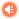•我转过几个弯 绕过那个小雨楼
•拿着蒲扇摆着衣衫渡着紧箍咒
•不问天涯不停留 喝过几壶酒
•不过年少白头道义放胸口
•倘若明天之后 遥看前尘剑封侯
•似那天上神仙无所求
•朝朝暮暮君如梦醒十分不为何理由
•是真是假是惶恐是无休
•路过这风雨花满楼 片刻都不停留
•我本这书生进京赶考留下许多愁
•你问有没有时候 我叹这天道默悠悠
•能否与我一醉方休
•又过了几个弯 算尽天量道莫慌
•踏这田园闻这芳草香
•跌跌撞撞仗剑天涯折煞不枉无笔良
•是梦是幻是温柔是家乡
•路过这风雨花满楼 片刻都不停留
•我本这书生进京赶考留下许多愁
•你问有没有时候 我叹这天道默悠悠
•能否与我一醉方休
•路过这风雨花满楼 片刻都不停留
•我本这书生进京赶考留下许多愁
•你问有没有时候 我叹这天道默悠悠
•能否与我一醉方休
•谁能与我一醉方休

# JS 封装CSS选择器式的获取元素方法

``\$('#er span i').css('color','red')``

html代码如下：

``````<p id="er">
<span id="ps">span</span>
<span>span</span>
</p>``````

``````//调用Base
var \$ = function(args) {
return new Base(args);
}
//基础库
function Base(args) {
this.arr = [];
if(args.indexOf(' ') != -1){

}else {

}
}``````

``````//调用Base
var \$ = function(args) {
return new Base(args);
}
//基础库
function Base(args) {
this.arr = [];
if(args.indexOf(' ') != -1){

}else {
//如果传入的值是文本
if(typeof args == 'string') {
if(args.charAt(0) == '#') {
this.arr.push(this.getId(args.substring(1)));
}
}else if(typeof args == 'object') {
//因为之前事件绑定时还传过this对象，这里做个兼容
if(args != undefined) {
this.arr = args;
}
}
}
}
//获取id元素
Base.prototype.getId = function(id) {
return document.getElementById(id);
}``````

``````//基础库
function Base(args) {
this.arr = [];
if(args.indexOf(' ') != -1){

}else {
//如果传入的值是文本
if(typeof args == 'string') {
switch(args.charAt(0)) {
case '#' :
this.arr.push(this.getId(args.substring(1)));
break;
case '.' :

break;
default :

}
}else if(typeof args == 'object') {
//因为之前事件绑定时还传过this对象，这里做个兼容
if(args != undefined) {
this.arr = args;
}
}
}
}``````

``````//基础库
function Base(args) {
this.arr = [];
if(args.indexOf(' ') != -1){

}else {
//如果传入的值是文本
if(typeof args == 'string') {
switch(args.charAt(0)) {
case '#' :
this.arr.push(this.getId(args.substring(1)));
break;
case '.' :
this.arr = this.getclass(args.substring(1));
break;
default :

}
}else if(typeof args == 'object') {
//因为之前事件绑定时还传过this对象，这里做个兼容
if(args != undefined) {
this.arr = args;
}
}
}
}

//获取class元素和id筛选
Base.prototype.getclass = function(className,parentNode) {
var node = null;
var temps = [];
if(parentNode != undefined) {
node = parentNode;
}else {
node = document;
}
var elements = node.getElementsByTagName('*');
for(var i = 0;i<elements.length;i++)  {
if(elements[i].className.match(className)) {
temps.push(elements[i]);
}
}
return temps;
}``````

``````//基础库
function Base(args) {
this.arr = [];
if(args.indexOf(' ') != -1){

}else {
//如果传入的值是文本
if(typeof args == 'string') {
switch(args.charAt(0)) {
case '#' :
this.arr.push(this.getId(args.substring(1)));
break;
case '.' :
this.arr = this.getclass(args.substring(1));
break;
default :
this.arr = this.getTagName(args);
}
}else if(typeof args == 'object') {
//因为之前事件绑定时还传过this对象，这里做个兼容
if(args != undefined) {
this.arr = args;
}
}
}
}

//通过元素名获取元素
Base.prototype.getTagName = function(tag,parentNode) {
var node = null;
var temps = [];
if(parentNode != undefined) {
node = parentNode;
}else {
node = document;
}
var tags = node.getElementsByTagName(tag);
for(var i = 0;i<tags.length;i++){
temps.push(tags[i]);
}
return temps;
}``````

getTagName 也是返回的数组，和上面一样this.arr = 返回的数组即可。

``````//基础库
function Base(args) {
this.arr = [];
if(args.indexOf(' ') != -1){
var childNodes = args.split(' ');
var temNodes = [];
var node = [];
for(var i = 0;i < childNodes.length;i++) {
if(node.length == 0) node.push(document);
switch(childNodes[i].charAt(0)) {
case '#' :
temNodes = [];
temNodes.push(this.getId(childNodes[i].substring(1)));
node = temNodes;
break;
case '.' :
temNodes = [];
for(var j = 0;j<node.length;j++) {
var temps = this.getclass(childNodes[i].substring(1),node[j]);
for(var k = 0;k<temps.length;k++){
temNodes.push(temps[k])
}
}
node = temNodes;
break;
default :
temNodes = [];
for(var j = 0;j<node.length;j++) {
var temps = this.getTagName(childNodes[i],node[j]);
for(var k = 0;k<temps.length;k++){
temNodes.push(temps[k])
}
}
node = temNodes;
}
}
this.arr = temNodes;
}else {
//如果传入的值是文本
if(typeof args == 'string') {
switch(args.charAt(0)) {
case '#' :
this.arr.push(this.getId(args.substring(1)));
break;
case '.' :
this.arr = this.getclass(args.substring(1));
break;
default :
this.arr = this.getTagName(args);
}
}else if(typeof args == 'object') {
//因为之前事件绑定时还传过this对象，这里做个兼容
if(args != undefined) {
this.arr = args;
}
}
}
}``````

temNodes也是push方法，这样就导致没啥效果啊，和this.arr.push一样，所以在temNodes.push之前要对这个数组重置。temNodes = [];

id部分好了，下面为class的时候

0
• 本文分类：JavaScript
• 本文标签：CSS选择器式的获取元素方法
• 流行热度：已超过 101 人围观了本文
• 最后更新：2018年12月2日 - 22时45分11秒
• 发布日期：2018年12月2日 - 22时45分11秒
• 版权申明：本文系作者@木灵鱼儿原创发布在木灵鱼儿 - 有梦就能远航站点。未经许可，禁止转载。